7961

Mathematics Algebra Level: Misc Level

Factor completely;

50a2- 40a + 8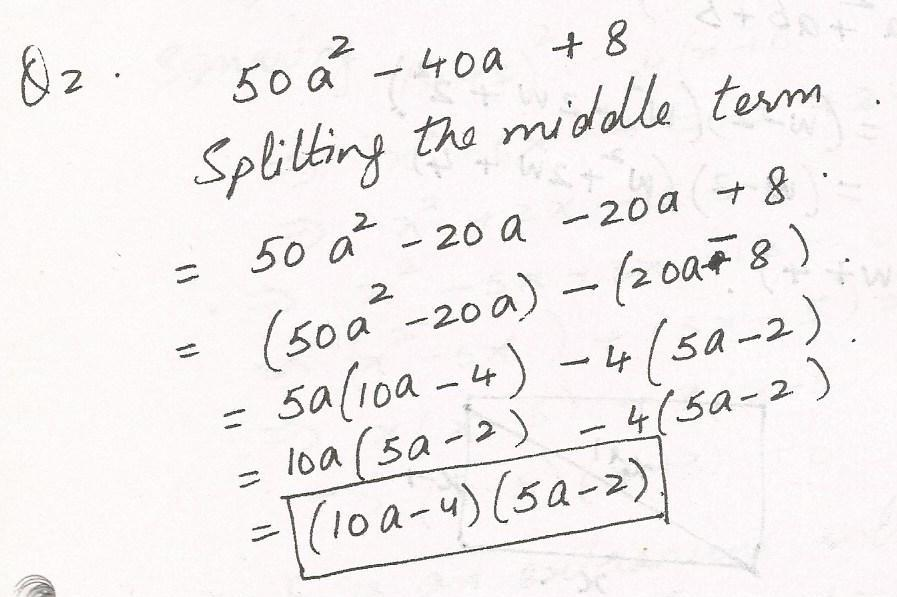7947

Mathematics Algebra Level: Misc Level

Solve for y; ax+dy=g

bx+cy=f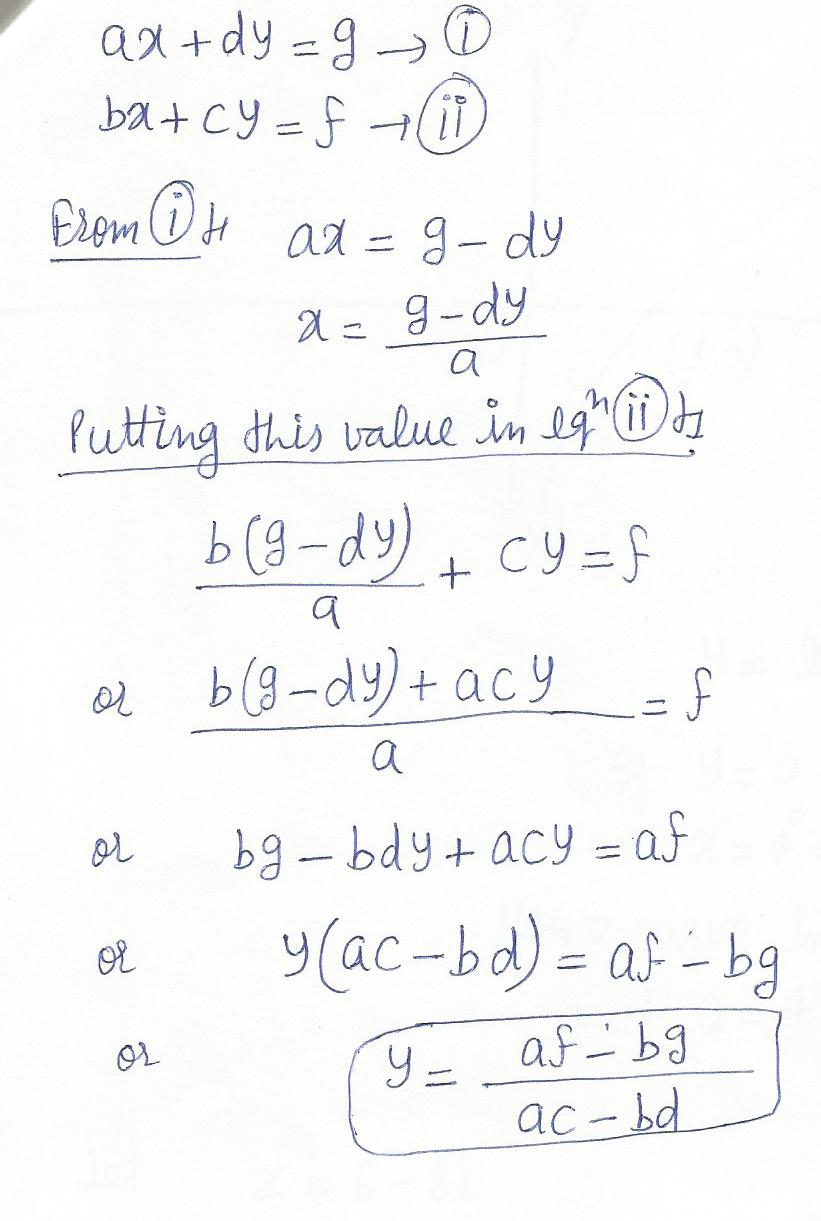7944

Mathematics Algebra Level: Misc Level

Solve for x

- 4 < (3x+6)/4 - (2x+7))/5  4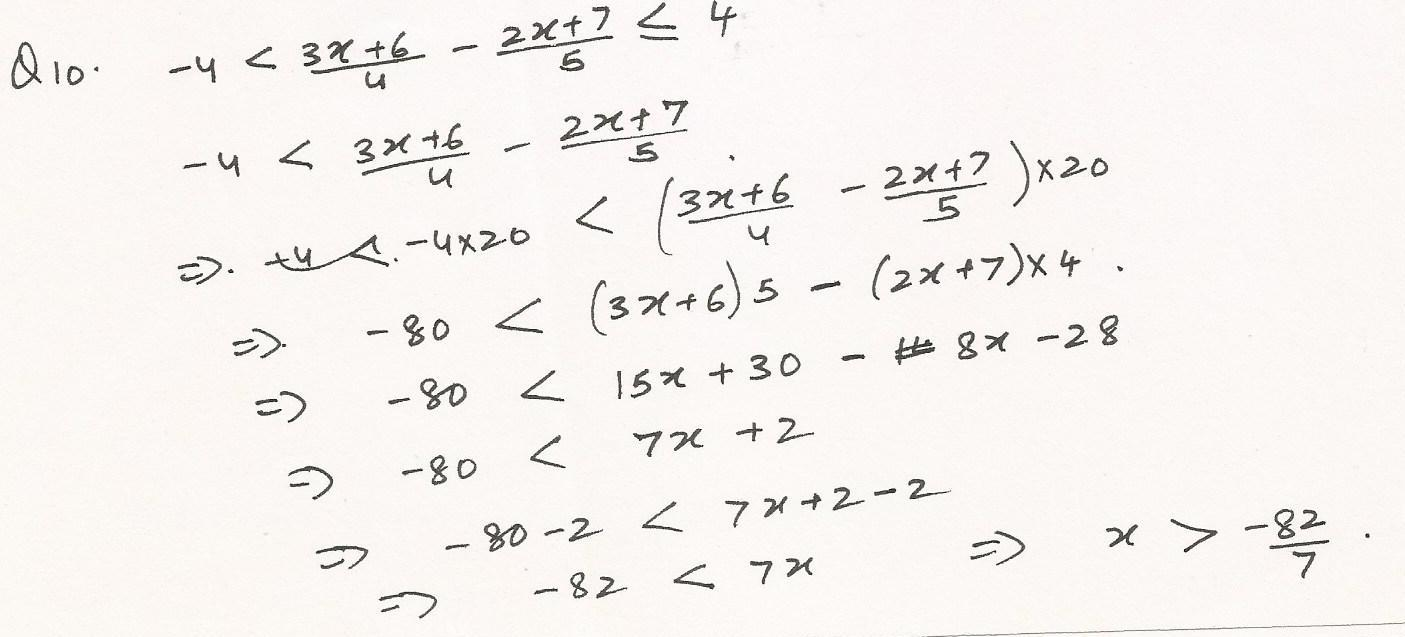7943

Mathematics Algebra Level: Misc Level

Solve for x

x/2 + (x-6)/3 + (x-3)/4 = 2x - 22/3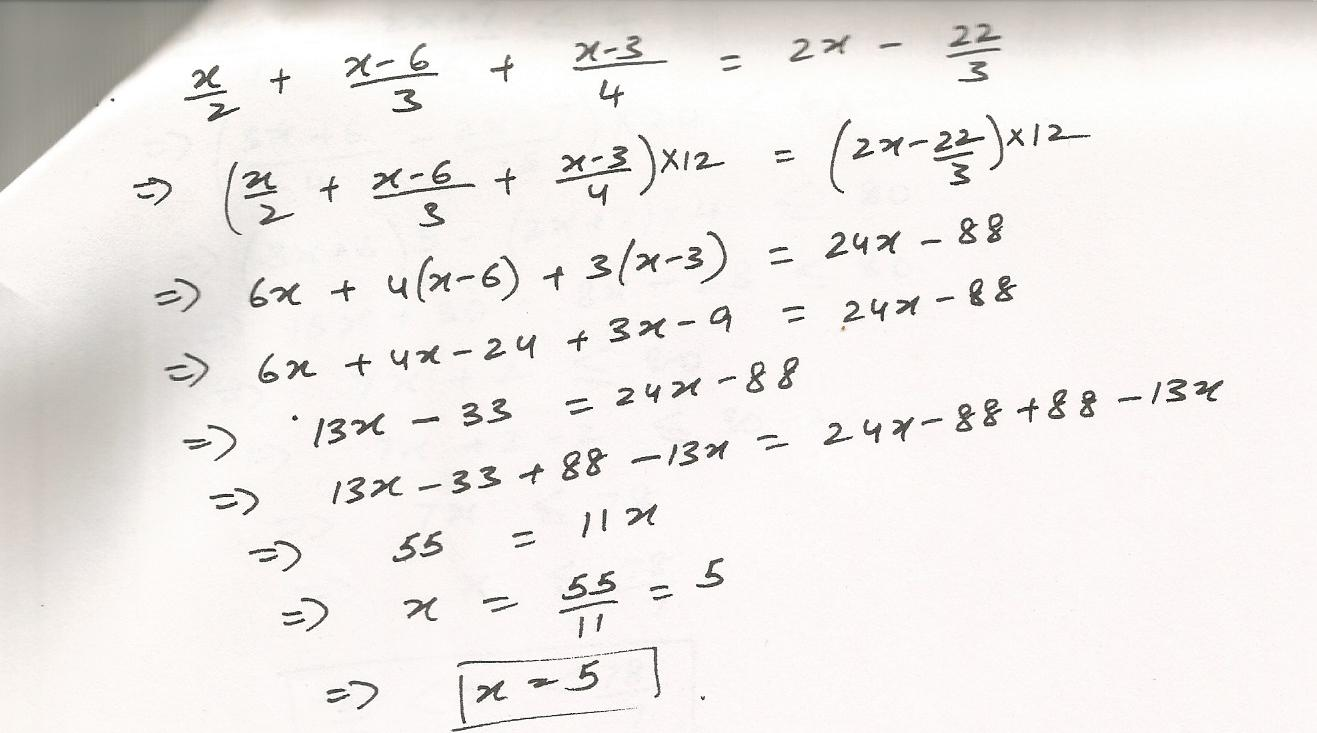7942

Mathematics Algebra Level: Misc Level

Solve for x

-27< -4X+5 < 21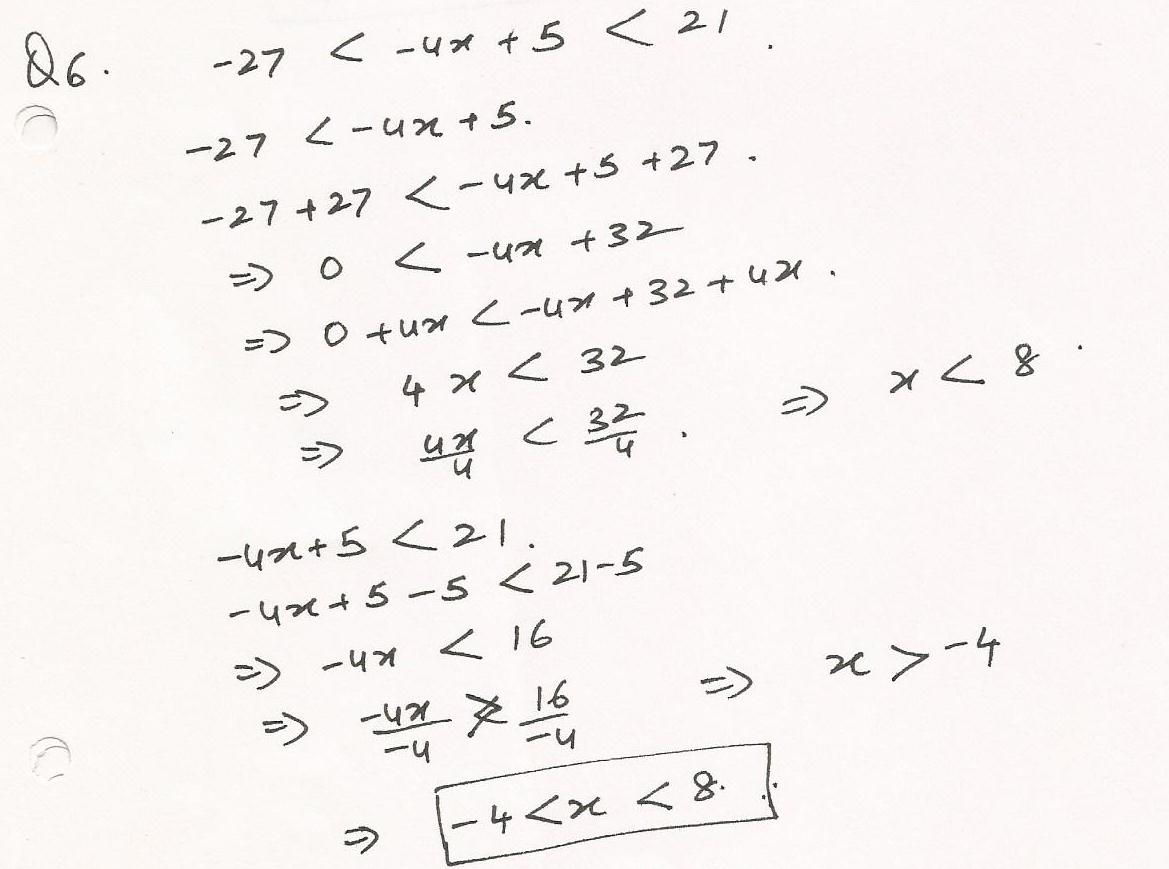7940

Mathematics Algebra Level: Misc Level

Solve for x

(x+7)/45 = (x+6)/42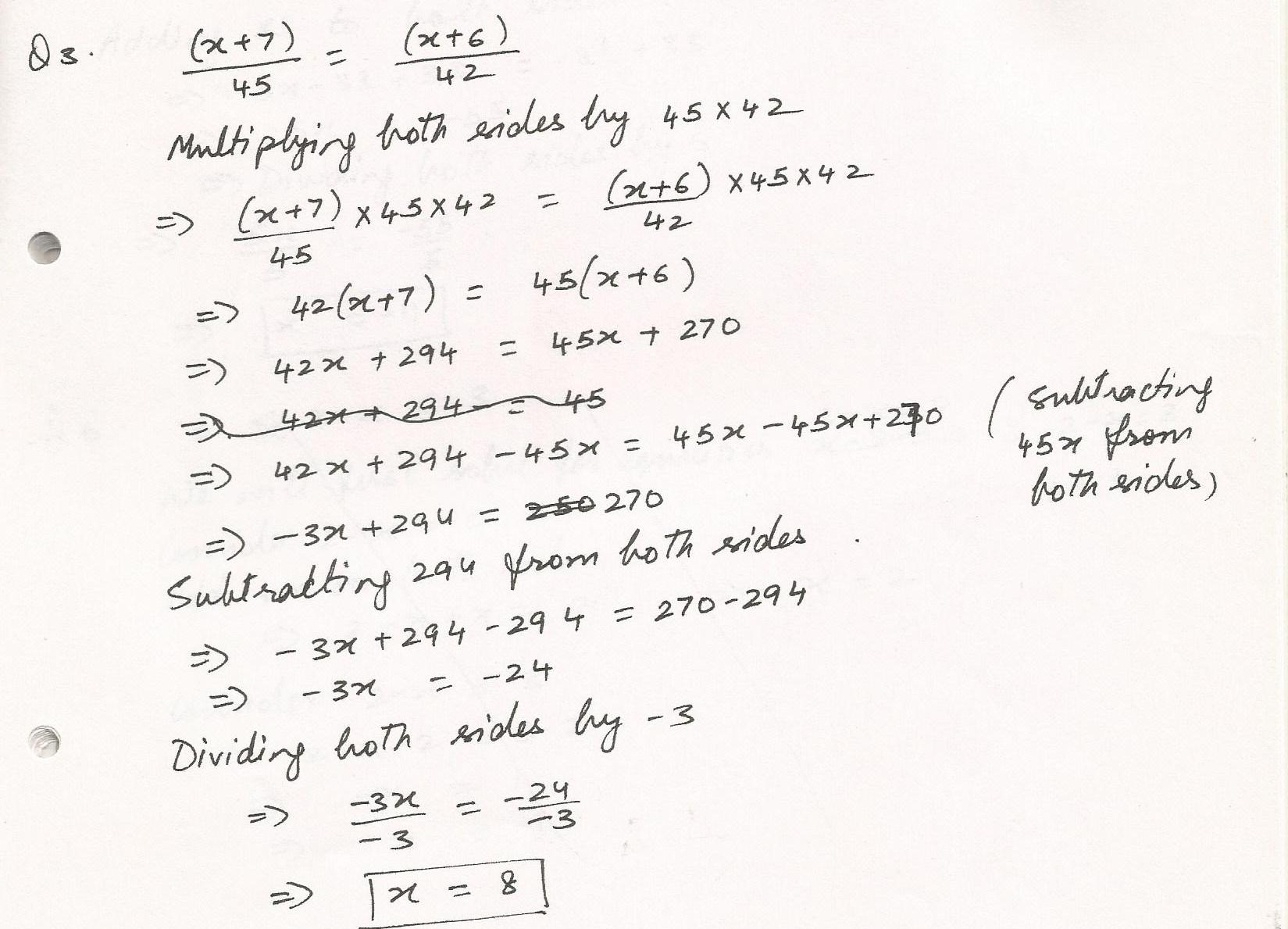7939

Mathematics Algebra Level: Misc Level

Simplify

(10X+3)/7 + (9X+2)/7 + 7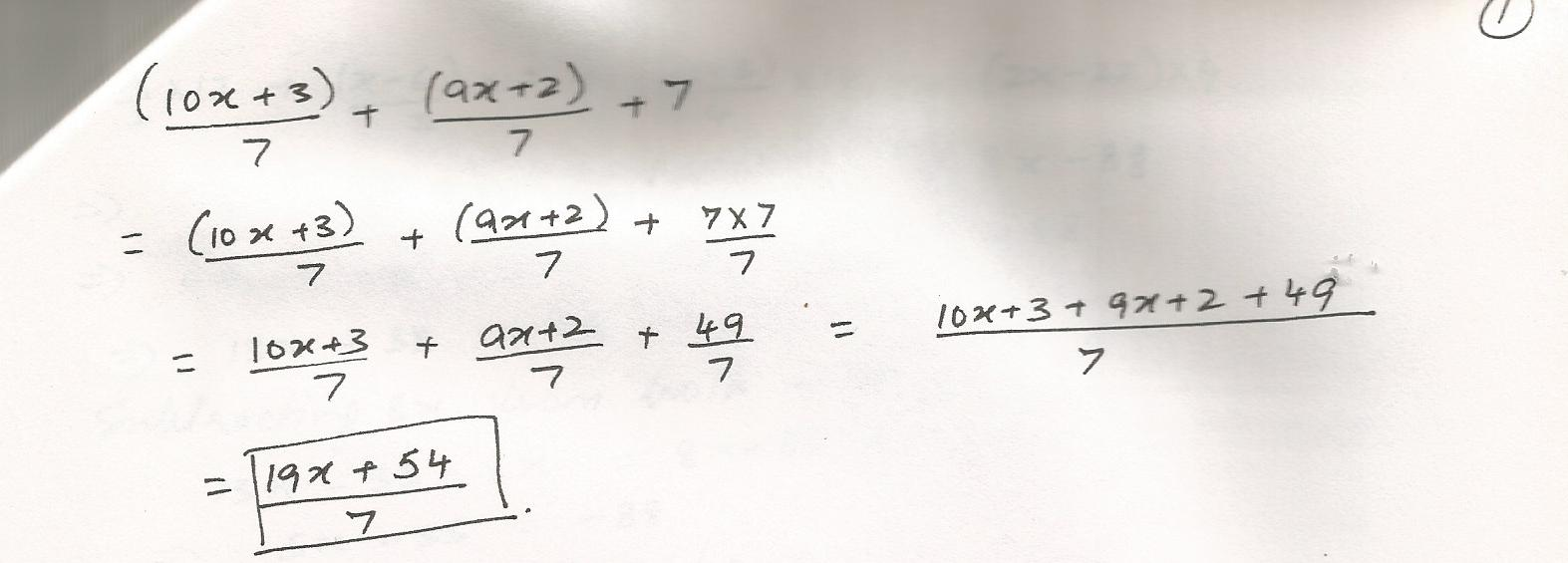6148

Mathematics Algebra Level: Misc Level

Factor x3-6X2+11x-6. One of the factor is (x-1)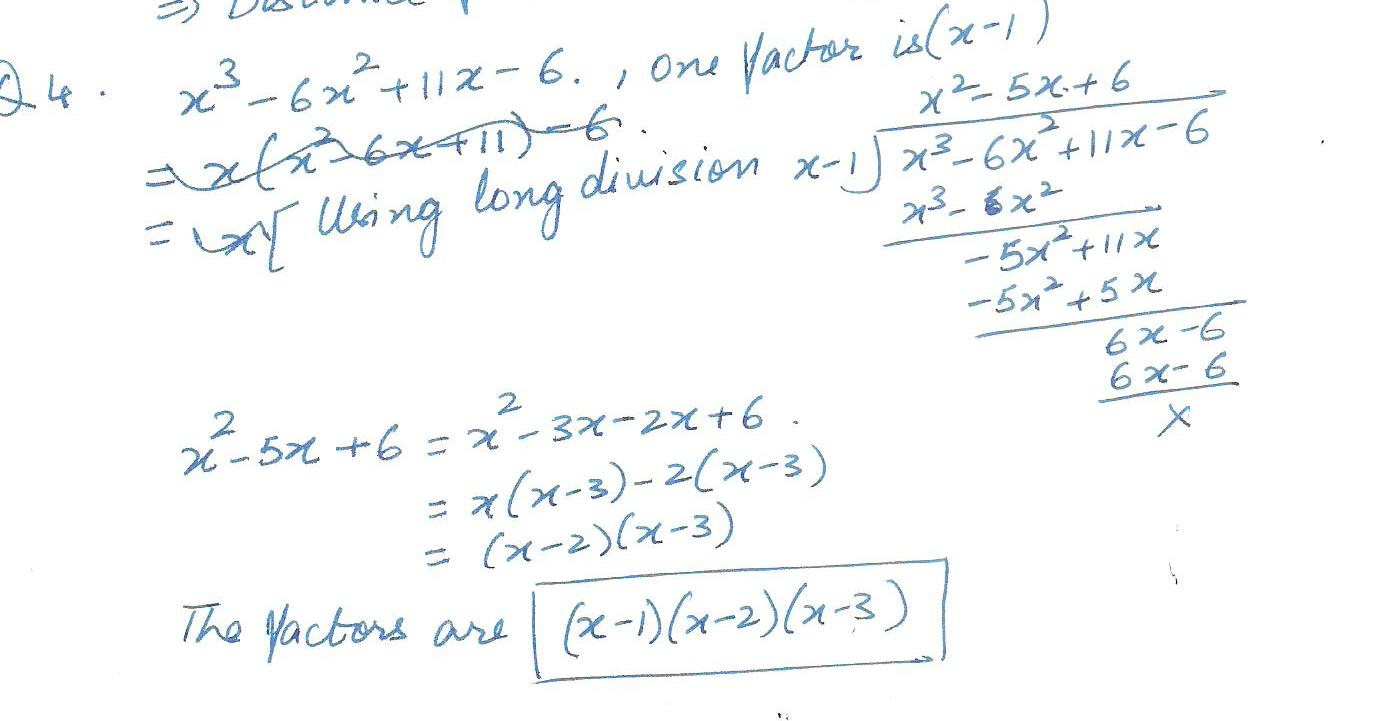6147

Mathematics Algebra Level: Misc Level

A 10- ft ladder is placed against a building so that the distance from the bottom of the ladder to the building is 2 feet less than the distance from the top of the ladder to the ground. What is the distance from bottom of the ladder to the building?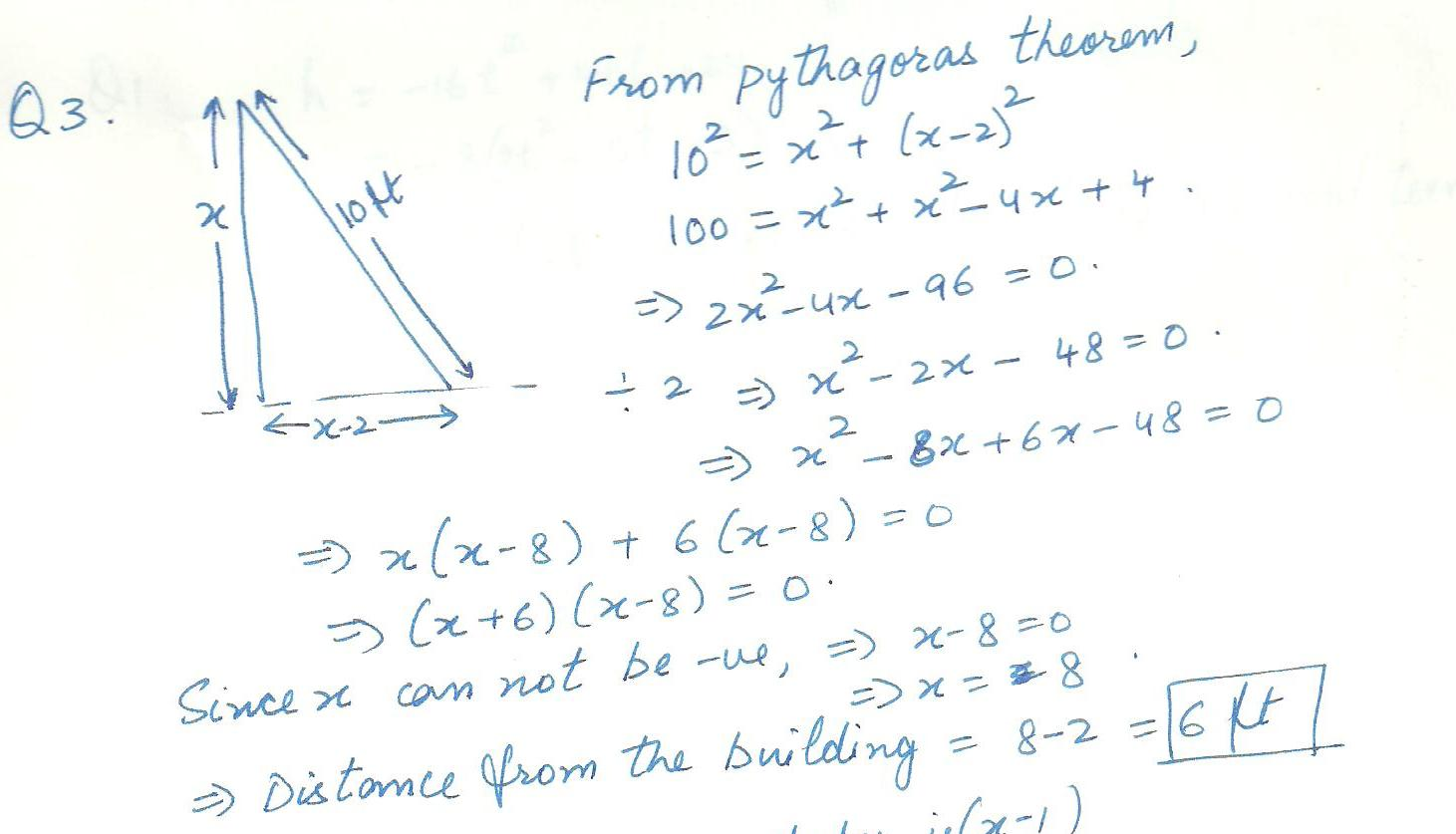6146

Mathematics Algebra Level: Misc Level

Find all positive and negative integers b for  which the following polynomial can be factored: 2x2+bx-15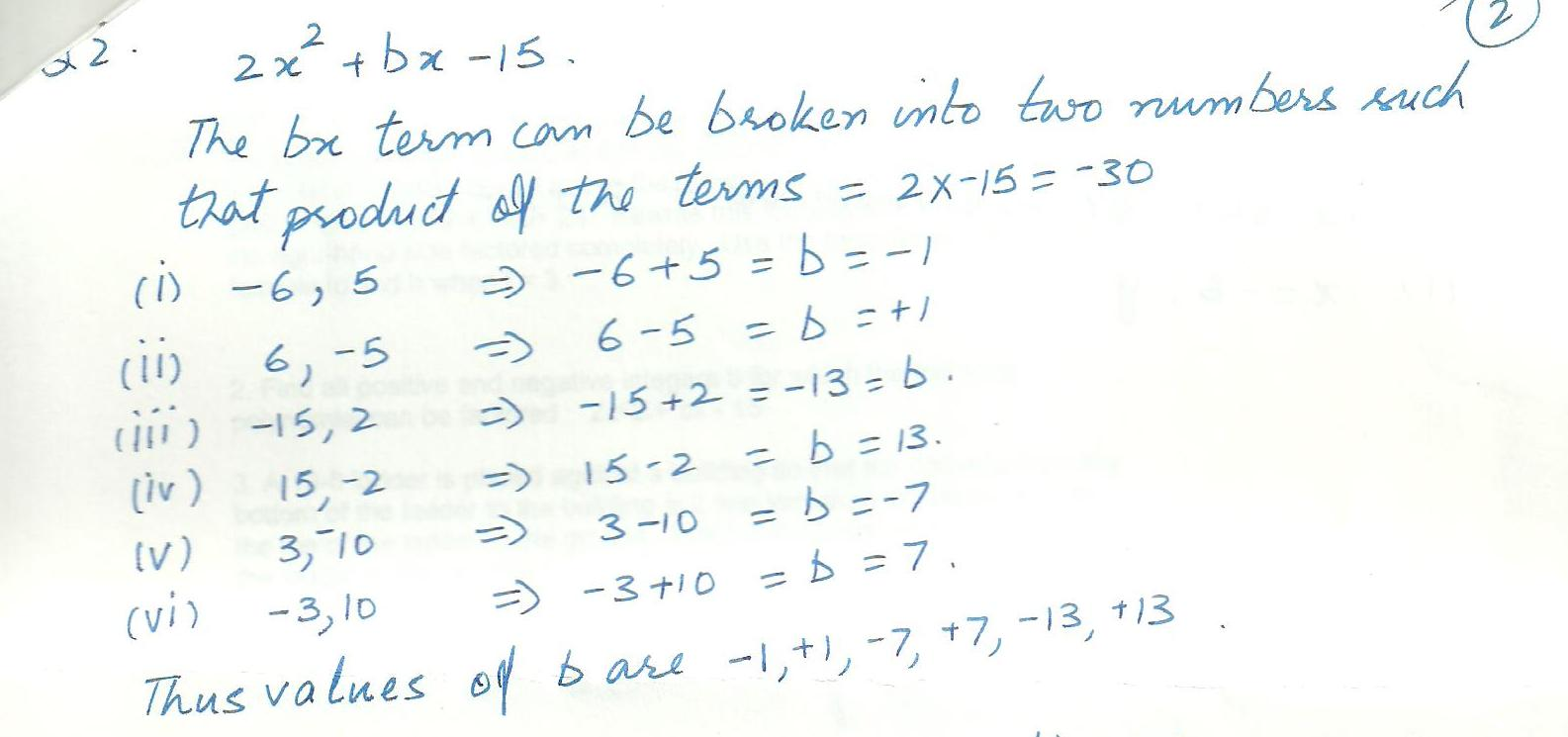6145

Mathematics Algebra Level: Misc Level

If a ball is thrown upward at 40 ft per second from a rooftop 24 ft above the ground , then its height above the ground t seconds after it is thrown is given by h=-16t2+40 t+24. Rewrite this formula with the polynomial on the right- hand side factored completely .Use the factored version of the formula to find h when t=3.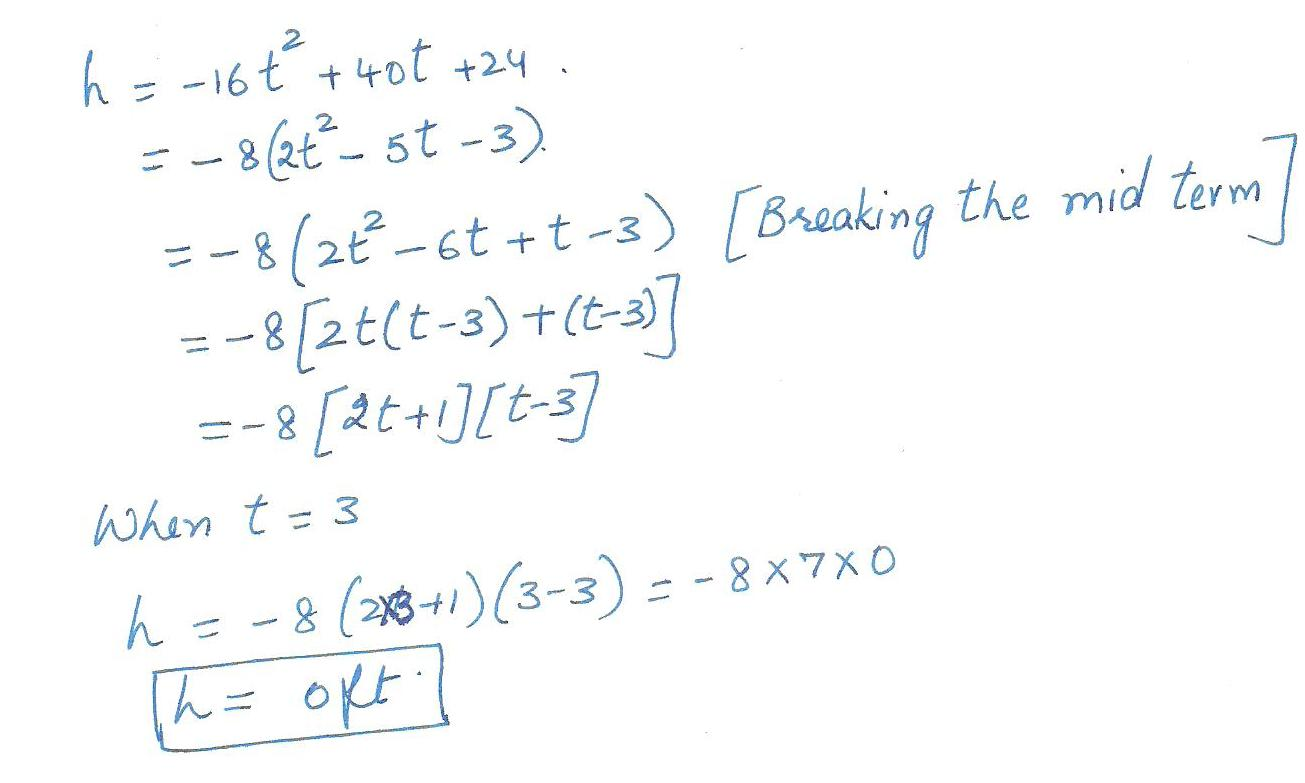4938

Mathematics Algebra Level: Misc Level

If r ={ 2z, 4 x, 6y } and s ={ x- z,2 x y,y +2 z } find r.s (r dot s) and rXs (r cross x)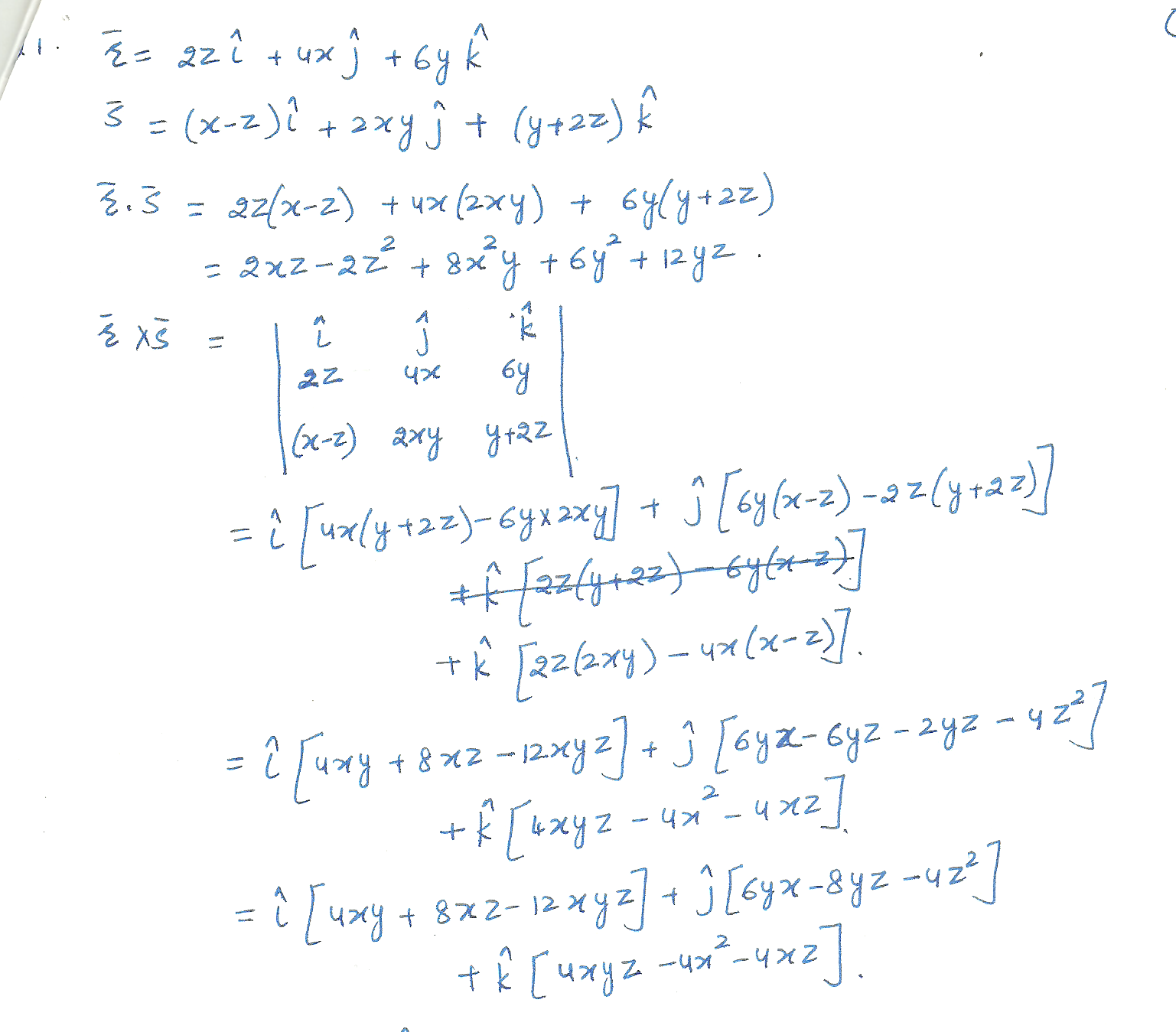4791

Mathematics Algebra Level: Misc Level

Solve the inequality. 33 < 4 x + 9 < 41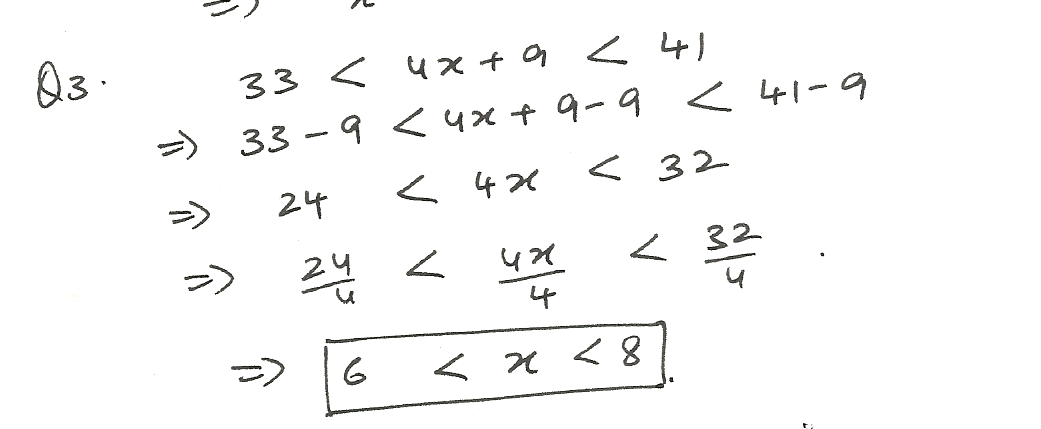4790

Mathematics Algebra Level: Misc Level

Solve the inequality. 4 ( x -7) < or =to 12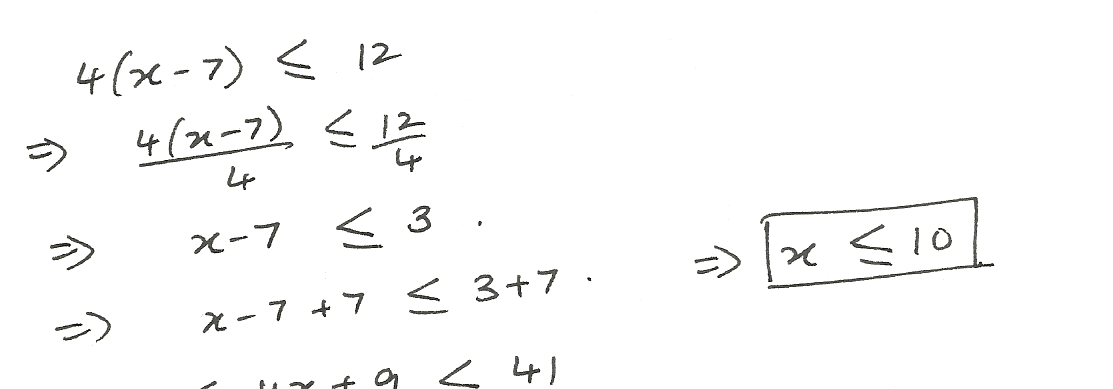4789

Mathematics Algebra Level: Misc Level

Solve the inequality. 9 x - 11 > or =to 4 x + 34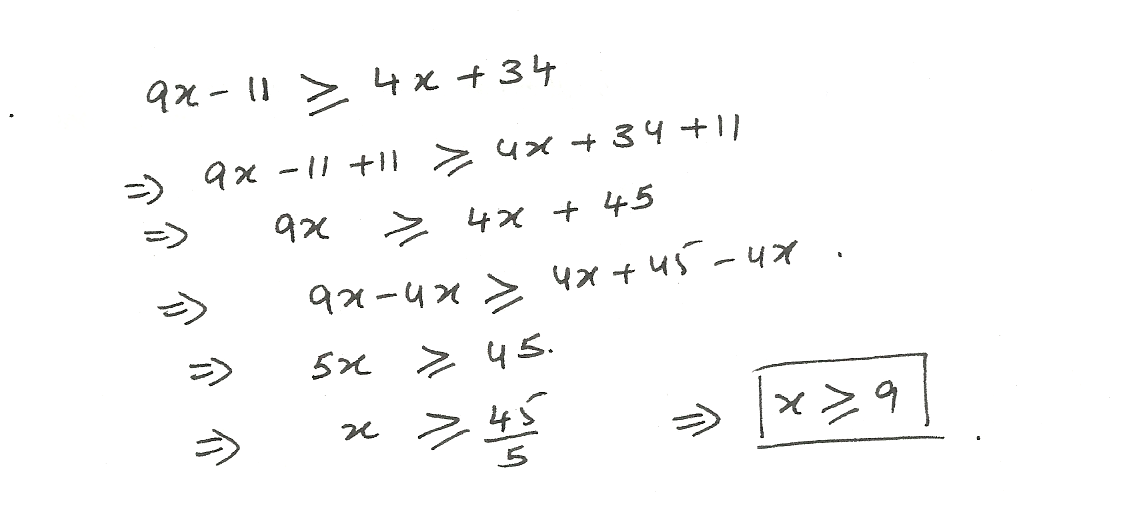Displaying 16-30 of 40 results.